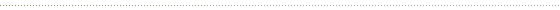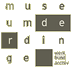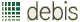# leonardo da vinci : the codex leicester

## how can one determine the weight of a ball in parts?

one must hang the ball, whose total weight has been ascertained before, on a rope. the hanging rope produces a perpendicular. now one hangs a known weight, which corresponds to a certain part of the weight of the ball, next to the ball, by which the ball will be knocked off the perpendicular. for example, if one wants to find a one-pound part of a three-pound heavy ball, one hangs a one-pound weight next to the ball. now one pound of the ball is hanging on one side of the perpendicular and two pounds of the ball are handing on the other side. the perpendicular thus defines the potential point of intersection for removing one part weighing one pound.### water